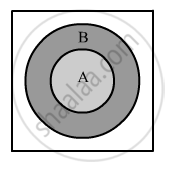Department of Pre-University Education, KarnatakaPUC Karnataka Science Class 11
Advertisement Remove all ads

# If a and B Are Two Sets Such that a ⊂ B Then Find: a ∪ B - Mathematics

If A and B are two sets such that $A \subset B$ then find:

$A \cup B$

Advertisement Remove all ads

#### Solution

From the Venn diagrams given below, we can clearly say that if A and B are two sets such that $A \subset B$

Form the given Venn diagram, we can see that $A \cup B$=BConcept: Venn Diagrams
Is there an error in this question or solution?
Advertisement Remove all ads

#### APPEARS IN

RD Sharma Class 11 Mathematics Textbook
Chapter 1 Sets
Exercise 1.5 | Q 1.2 | Page 21
Advertisement Remove all ads

#### Video TutorialsVIEW ALL 

Advertisement Remove all ads
Share
Notifications

View all notifications

Forgot password?